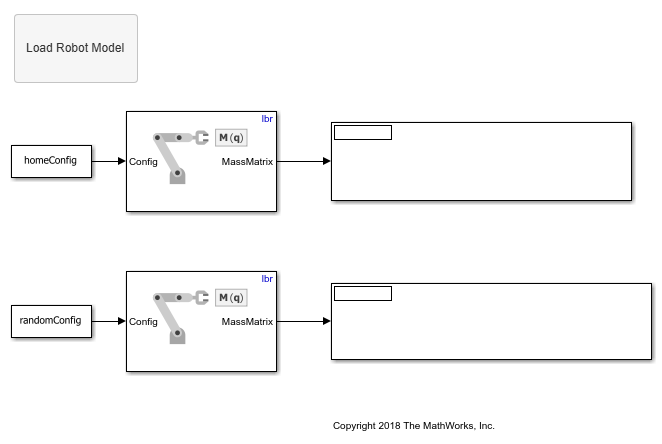Get Mass Matrix for Manipulators in Simulink

This example shows how to calculate the mass matrix for a robot manipulator using a rigidBodyTree model. In this example, you define a robot model and robot configurations in MATLAB® and pass them to Simulink® to be used with the manipulator algorithm blocks.

Load a RigidBodyTree object that models a KUKA LBR robot. Use the homeConfiguration functions to get the home configuration or home joint positions of the robot. Use the randomConfiguration function to generate a random configuration within the robot joint limits.

lbr.DataFormat = 'column';

homeConfig = homeConfiguration(lbr);
randomConfig = randomConfiguration(lbr);

Open the model. If necessary, use the Load Robot Model callback button to reload the robot model and configuration vectors.

The Joint Space Mass Matrix block calculates the mass matrix for the given configuration.

open_system('mass_matrix_example.slx')Run the model to display the mass matrices for each configuration.